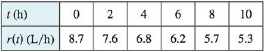Chapter 5.1, Problem 15E### Single Variable Calculus: Early Tr...

8th Edition
James Stewart
ISBN: 9781305270343

#### Solutions

Chapter
Section### Single Variable Calculus: Early Tr...

8th Edition
James Stewart
ISBN: 9781305270343
Textbook Problem

# Oil leaked from a tank at a rate of r(t) liters per hour. The rate decreased as time passed and values of the rate at two-hour time intervals are shown in the table. Find lower and upper estimates for the total amount of oil that leaked out.To determine

The lower estimate and upper estimate for the total amount of oil that leaked out.

Explanation

Given:

The function r(t) is an decreasing function.

The number of intervals is n=5.

Each interval value is Δt=2h.

Calculation:

The distance approximation R5 provides the lower estimate for the total amount of oil leaked from the tank and L5 provides the upper estimate for the total amount of oil leaked from the tank.

The expression to find the lower estimate for the total amount of oil leaked (Rn) is shown below:

Rn=r(t1)Δt+r(t2)Δt+...+r(tn)Δt (1)

Here, for the time t1, the rate is r(t1), the time interval is Δt, for the time t2, the rate is r(t2), and for the time tn, the rate is r(tn).

Substitute 5 for n in Equation (1).

R5=r(t1)Δt+r(t2)Δt+r(t3)Δt+r(t4)Δt+r(t5)Δt (2)

Substitute 7.6L/h for r(t1), 2h for Δt, 6.8L/h for r(t2), 6.2L/h for r(t3), 5.7L/h for r(t4), and 5.3L/h for r(t5) in Equation (2).

R5=(7.6×2)+(6.8×2)+(6.2×2)+(5.7×2)+(5.3×2)=15

### Still sussing out bartleby?

Check out a sample textbook solution.

See a sample solution

#### The Solution to Your Study Problems

Bartleby provides explanations to thousands of textbook problems written by our experts, many with advanced degrees!

Get Started

#### Proof Prove that lnxy=ylnx.

Calculus: Early Transcendental Functions

#### In problems 23-58, perform the indicated operations and simplify. 39.

Mathematical Applications for the Management, Life, and Social Sciences

#### Sometimes, Always, or Never: If {an} is increasing and bounded above, then {an} converges.

Study Guide for Stewart's Single Variable Calculus: Early Transcendentals, 8th